# Calculation of timber in a cube: formulas and tables

The need for calculating lumber rises before each developer. A person who does the work with his own hands, the calculation of the number of boards, cant or brick determines the cost of building materials. A person who hired workers and provided professionals to decide how much and what to purchase in order to build a building would be interesting to check the master’s calculations with his calculations. We give different ways to calculate lumber in one cube. You will need a construction tape measure and a pencil with a calculator.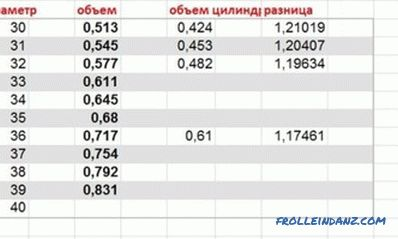Table of calculation of the cubic capacity of logs.

## Method 1: mathematical calculations and formulas

The calculation of sawn timber in a cubic meter of space can be performed using well-known formulas (from a school mathematics course). The volume of a rectangular shape (parallelepiped) is equal to the product of its three parameters: length, width, and height.

V = a b c h Figure 1.

The board or bar is a parallelepiped. The length, width and height of a cut tree can be measured with a building tape measure. Their sizes are equal in cross section (in contrast to unedged lumber, the calculations of which will be given below). Therefore, we will calculate the volume of one wooden product.

Length: a = 6.1 m.

Width: b = 20 cm.

Thickness: c = 2.5 cm.

We translate The obtained values ​​are in general units (meters).

Length is the same: a = 6.1 m.

Width: b = 0.2 m.

Thickness: c = 0.025 m.

We perform calculations:

V = 6.1 x 0.2 x 0.025 = 0.0305 m3.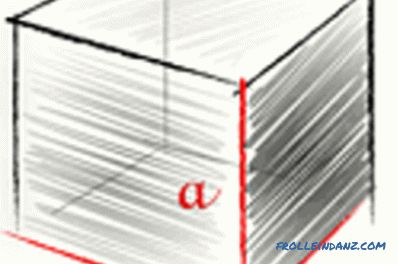Image 1. Calculate sawn timber in a cubic meter using the formula: V = a b c h.

The resulting volume is a digit with several digits after the decimal point. There is a desire to round the value, converting 0.0305 to 0.031 m3. This is what most sellers do. On two, three, ten pieces this rounding is hardly noticeable. The tree rises in price on kopeks in comparison with the present, actual cost.

If the calculations are carried out in large-scale construction (a house from a bar, the erection of a wooden frame under the roof), here the amount of the seller’s building allowance is supposed to be expressed in a more significant figure in order to simplify.

For example, you acquire a hundred of such floorboards (the actual volume of which is equal to 0.0305 m3, but in the opinion of the seller it is assumed to be 0.031 m3).

The difference of material for one floorboard is:

0.031 - 0.0305 = 0.0005 m3.

For a hundred floorboards, this difference increases to 0.05 cubic meters, which you do not purchase, but pay to the seller. For a thousand boards it is already 0.5 cubes.

Now back to determining the amount of your sawn timber in a cube. To find out how much of the plank material contains a cubic meter of space, divide 1 cube by the volume of one floorboard (obtained value V).

1: 0.0305 = 32.459 pieces.

The resulting number is also not an integer. Here rounding may be to your advantage. A value of 32.459 is rounded up to 32.5 or up to 33 boards in a cube.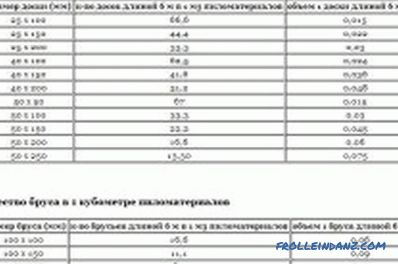Figure 2. Table of the number of boards in a cubic meter.

If you make a large volume of purchases of various lumber, the table will make it easier for you to determine the amount of materials for each type of tree you are purchasing. To be sure that the payment is in accordance with the amount of the tree being purchased, we will check one of the table values ​​with our calculations.

Example. In the selected floorboard (in which we measured the parameters previously) the length exceeds the required standard by 10 cm (standard length of sawn timber is 6 m). Therefore, technological surcharges of length of 10 or 20 cm are not accepted for calculations. As a result, the tabular figure for the number of boards (25 x 200 mm in size) is 33 pieces. Image 2.

## Method 3: for unedged lumber

Unedged boards differ in different widths. It can be 30 cm from one edge, and narrowed to 15 or 20 cm from the other end. How does the amount of building material in a cubic meter be calculated and how to calculate its cost?

The average width of wood is taken in the calculations of uncut materials products. Measure the widest place, the narrowest and reduce their mathematical values ​​to the average. For example:

The width of the larger edge is 32 cm. For another, the width is smaller and is 18 cm (thickness is 25 mm, the standard length is 6 m). Perform the following mathematical operations:

Average width b = (32 + 18): 2 = 25 cm (250 mm for table determination or 0.025 m in performing your own calculations).

Next, you can use the table above for trimming material. Since there are no 250 x 25 mm parameters in it, we will use the value of 250 x 50 mm (it will be exactly twice as large as the thickness of 50 mm is twice as thick as ours).For a thickness of 50, the amount of material in a cube will be 13.3 pieces. So for our size, the number of unedged boards (25 mm thick) will be

13.3: 2 = 6.15 pieces.

Here is such an interesting building mathematics.

•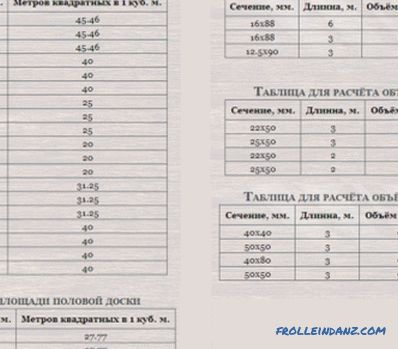Detailed calculation of the cubature of the cut board

Calculation of the cubature of the edged board. What is a cubicle and what elements are included in it. Why it is better to make all the calculations in my head. Calculation of material with a set of popular parameters.

•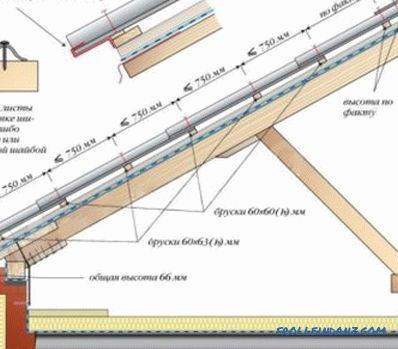How to choose the distance between the rafters?

The distance between the rafters, taking into account the slope of the roof and roofing material. The method of calculation and example calculations. Rafter system for a metal tile, a professional flooring, an ondulin.